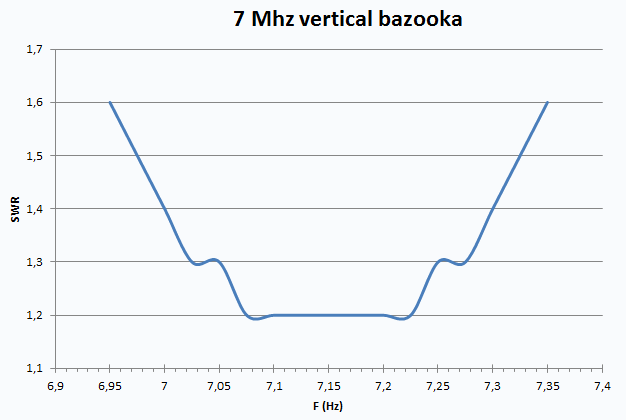I needed a omnidirectional antenna for the 40m.
Here is the schema of the antenna :The design formulas are quite simple :

A = 230/F (feet)
B = A * Vf
C = (A - B)
S = 4"
R = A * 1.05

## Dimensions in meters

A = (230/F)*0.305 (meters)
B = A * Vf
C = (A - B)
S = +/- 10cm
R = A * 1.05

The angle of the radials will be idealy between 35° and 45°

Vf (velocoty factor of used coax) depends of the type of cable. Don't trust to much the  clasic 0.66 value : the best is to mesure the Vf with a antenna analyser, results are some surprising (espacialy when you use old coax cables).

Applied as there are, those formulas gived me results close to what theoreticaly expected.No re-tuning was necessary.